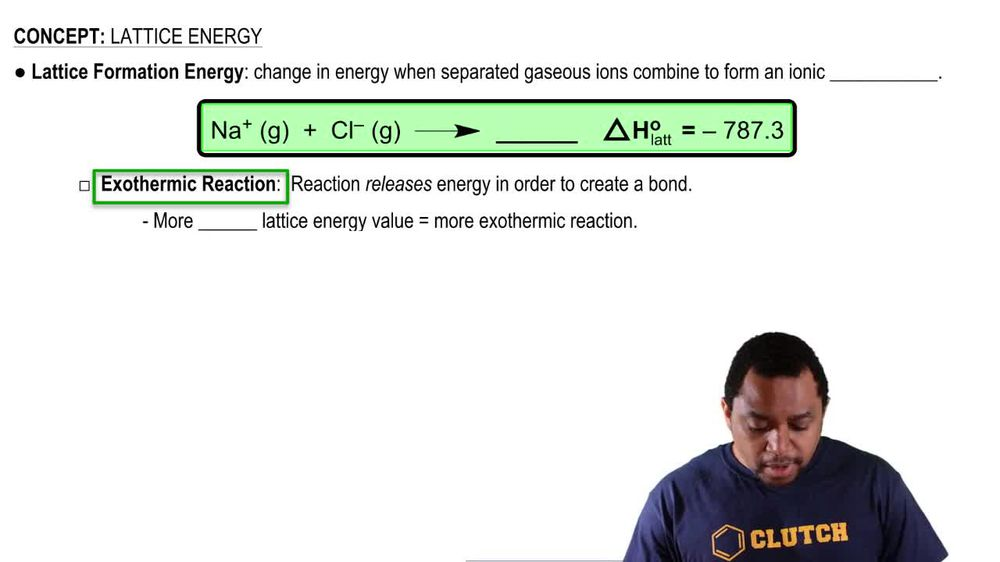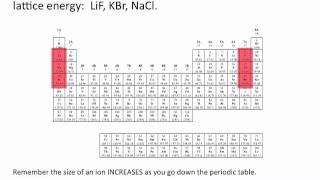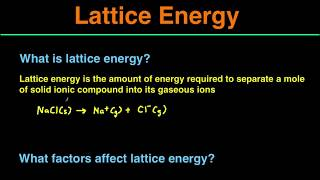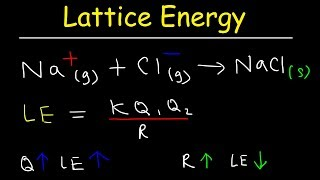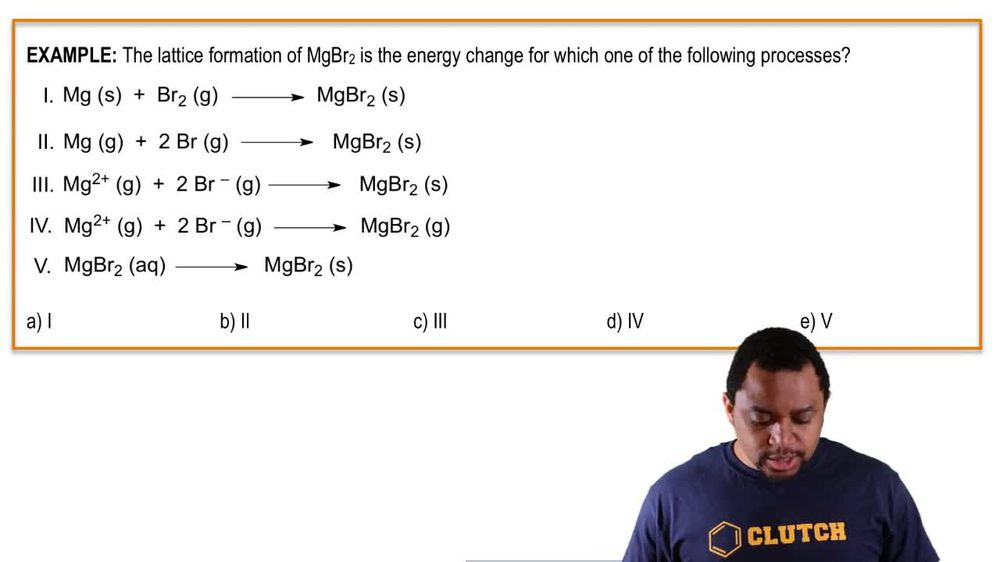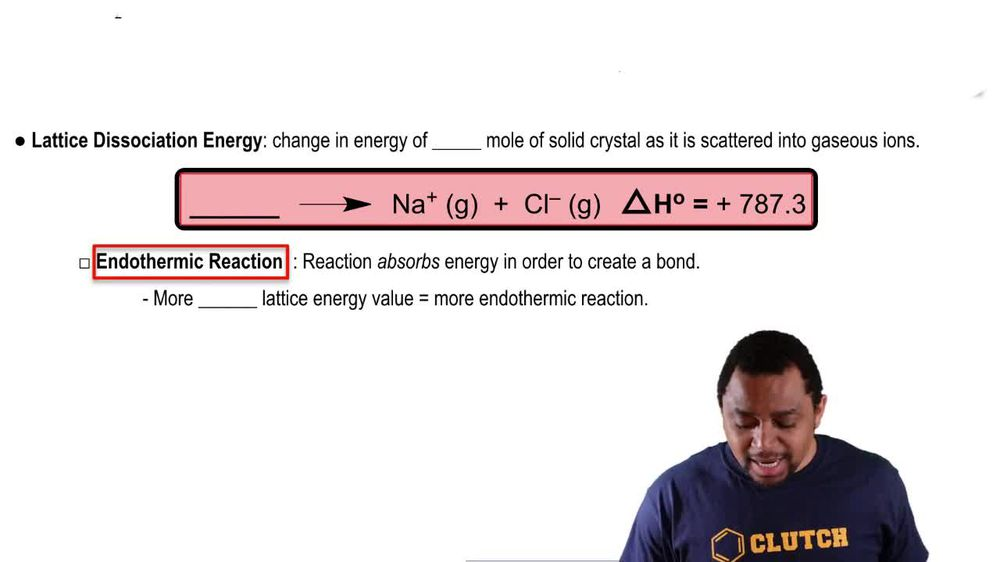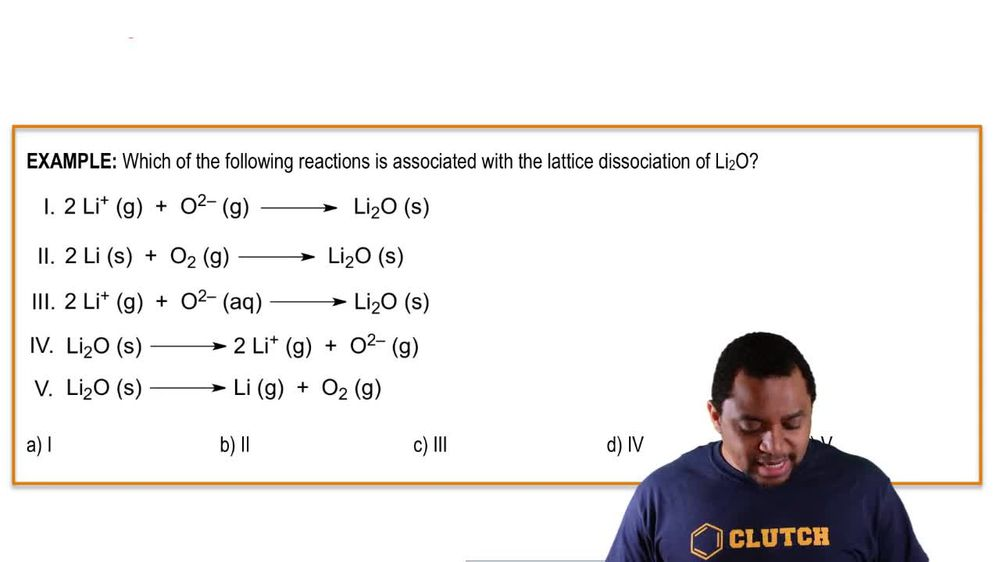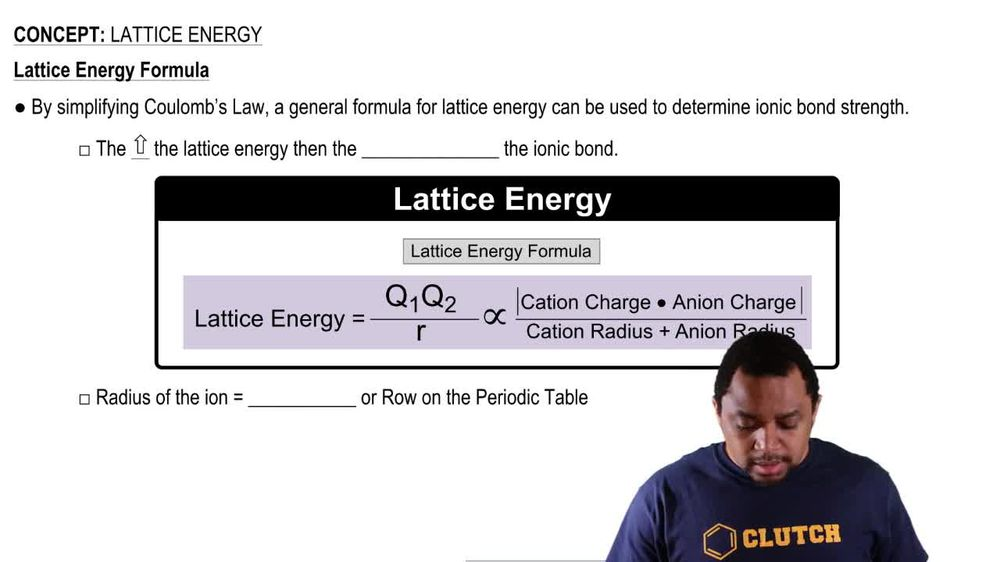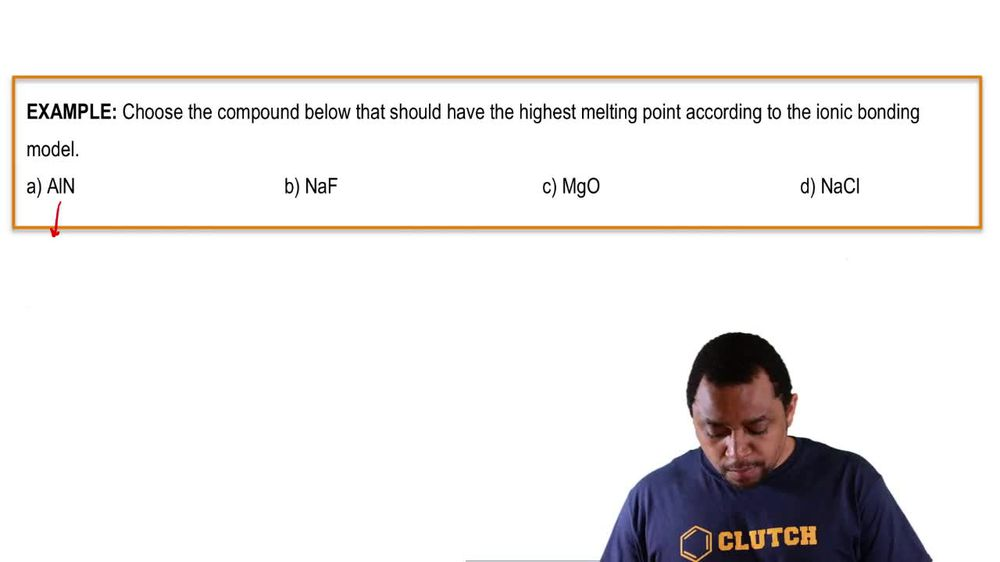Start typing, then use the up and down arrows to select an option from the list.
1. 11. Bonding & Molecular Structure2. Lattice Energy# Lattice Energy Example 4

by Jules Bruno
72 views
0
here. It says, choose the compound below. That should have the highest melting point according to the Ionic bonding model. So remember, highest melting point means highest lattice energy. When you break these all up into their ions, based on the groups that they're found in, we'll be able to figure out the charge here. Remember group number and their charges. Come now, we're gonna say lattice energy for all of them. In absolute brackets, we have their charges multiply together on the bottom. We're going to have the periods that they're founded. So here all I'm doing is I'm in putting the charges for each one. So remember, that's the first part we need for lattice energy. So here we're gonna multiply their charges in absolute terms. So for all of them, the number will be positive. Now, take the periods or rose that they found on the periodic table, and they're gonna go on the bottom, but they're added together. So this would be three plus two. This would be three plus 23 plus two and three plus three. Now we're going to bring everything down. So this is 9/5. This is 1/5 this is 4/5, and finally, this one here is 1/6. The one with largest lattice energy is aluminum nitride, and because of that, it will have the highest melting point. So just remember the higher lattice energy, the higher your melting point, the higher your boiling point. And remember, the lower your solid ability. I know the question doesn't talk about boiling point in Saudi ability, but it's important to remember these relationships.